+

# Solving Linear Inequalities

Author: Sophia Tutorial
##### Description:

Solve a linear inequality with one variable.

(more)

Sophia’s self-paced online courses are a great way to save time and money as you earn credits eligible for transfer to many different colleges and universities.*

No credit card required

37 Sophia partners guarantee credit transfer.

299 Institutions have accepted or given pre-approval for credit transfer.

* The American Council on Education's College Credit Recommendation Service (ACE Credit®) has evaluated and recommended college credit for 32 of Sophia’s online courses. Many different colleges and universities consider ACE CREDIT recommendations in determining the applicability to their course and degree programs.

Tutorial
what's covered
This tutorial covers solving linear inequalities, through the definition and discussion of:
1. What Is an Inequality?
2. Operations in Inequality Statements
3. Solving an Inequality

## 1. What Is an Inequality?

An inequality is a mathematical statement that two quantities are not equal in value. You can use inequality symbols to show that one quantity is greater than or less than another quantity. The following outlines the different inequality symbols and their meanings:Inequalities are used in statistics, business, economics, and optimization when comparing values to each other.

term to know
Inequality
A mathematical statement that two quantities are not equal in value

## 2. Operations in Inequality Statements

Just like with equations, you can add or subtract a value on both sides of an inequality and keep the inequality statement true.

EXAMPLE

If you have the inequality 3 is less than 5, and you add 2 to both sides, you have 3 plus 2 is less than 5 plus 2. This simplifies to 5 is less than 7, which is still a true statement.You can also multiply or divide by a positive number on both sides and keep the inequality statement true.

EXAMPLE

If you have the inequality 6 is greater than or equal to 4, and you multiply by 3 on both sides, you have 3 times 6 is greater than or equal to 3 times 4. This simplifies to 18 is greater than or equal to 12, which is still true.big idea
However, it is important to note that if you multiply or divide by a negative number on both sides of the inequality, the statement becomes untrue until you flip or reverse the inequality sign.

EXAMPLE

If you have the inequality 6 is greater than or equal to 4, and you multiply by a negative 3 on both sides, you have negative 3 times 6 is greater than or equal to negative 3 times 4, which simplifies to negative 18 is greater than or equal to negative 12. Clearly, this is an untrue statement until you flip the sign. When you flip the sign, you now have negative 18 is less than or equal to negative 12, which is a true statement.## 3. Solving an Inequality

You may recall that in solving an equation, you isolate the variable using inverse operations. To isolate the variable, inverse operations are used to get all terms involving the variable on one side of the equation and all other terms to the other side of the equation.

The process for solving an inequality follows the same rules. You use inverse operations to isolate the variable, and what is done on one side of the inequality must be done on the other.

hint
Remember, the important difference in solving an inequality is that when you multiply or divide by a negative number, you have to flip the inequality sign.

### IN CONTEXT

Suppose Marcus has accepted a job selling cell phones. He will be paid \$1,500 plus 15% of his sales each month. He needs to earn at least \$2,430 per month to pay his bills. What amount of sales does Marcus need each month to be able to pay his bills?

Let the variable x represent the unknown quantity, which is the amount of sales Marcus needs each month. You know that 1,500 is the amount of Marcus's base monthly salary. You also know that Marcus earns an additional 15%, or 0.15, of his total sales each month. The combination of his base salary and his percentage of total sales has to be greater than or equal to 2,430, the amount of his bills. Therefore the inequality becomes: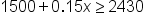Our inequality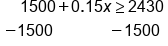Start by subtracting 1500 on both sides to isolate your x-variable.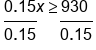Next, divide both sides by 0.15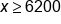Our solution

You can show this solution on a number line and use it to check your answer. If you pick a value in the highlighted range below, it should satisfy your original inequality. For example, if you pick the value 6,500 and substitute it back into your inequality, you have: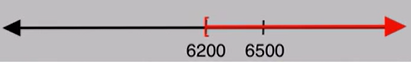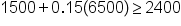Simplify the expression, starting with multiplication and moving on to addition, which gives you the inequality 2,475 is greater than or equal to 2,430, which is a true statement.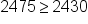try it
The following example involves solving an inequality that requires dividing by a negative number. Suppose Wei has a job paying \$25,000 a year and expects a raise of \$1,000 each year. Jaime has a job paying \$19,000 and expects to receive a raise of \$1,500 each year. When will Jaime be making more than Wei?
You want to determine how many years it will take for Jaime to make more than Wei, so the variable t can represent the number of years.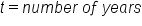You know that Wei starts at \$25,000 and gets a raise of \$1,000 for each year t. Jaime starts at \$19,000 and gets a raise of \$1,500 for each year t. Since you want to know when Jaime will make more than Wei, your inequality becomes 25,000 plus 1,000t is less than 19,000 plus 1,500t. The left side of the inequality represents the amount that Wei makes, and the right side of the inequality represents how much Jaime makes.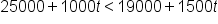Our inequality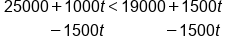Start by isolating the valuable on one side. Subtract 1500t from both sides of the inequality.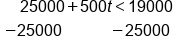Next, subtract 25000 from both sides of the inequality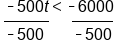Finally, divide by negative 500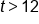Since we are dividing by a negative number, we must flip the inequality sign. t is greater than 12 is our answer. Jaime will make more than Wei after 12 years.

Again, you can show the solution on a number line, and if you pick a value in the highlighted range it should satisfy your original inequality. For example, if you pick the value 15 and substitute it back into your original inequality for t, you have: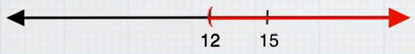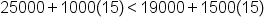Simplify with multiplication on both sides of the inequality, and you have 40,000 is less than 41,500, which is a true statement.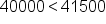summary
Today you learned about inequality symbols, including less than, less than or equal to, greater than, and greater than or equal to. You learned that when solving an inequality, you follow the same steps as when solving an equation to isolate the variable. However, you also learned that when solving an inequality, it is important to remember that the inequality symbol must be flipped when you multiply or divide by a negative number, in order to maintain a true statement.

Source: This work is adapted from Sophia author Colleen Atakpu.

Terms to Know
Inequality

A mathematical statement that two quantities are not equal in value.

Rating